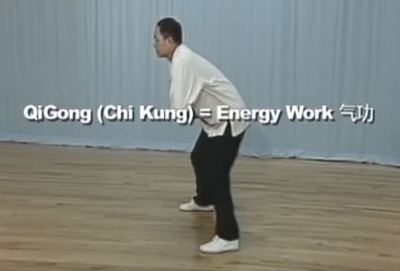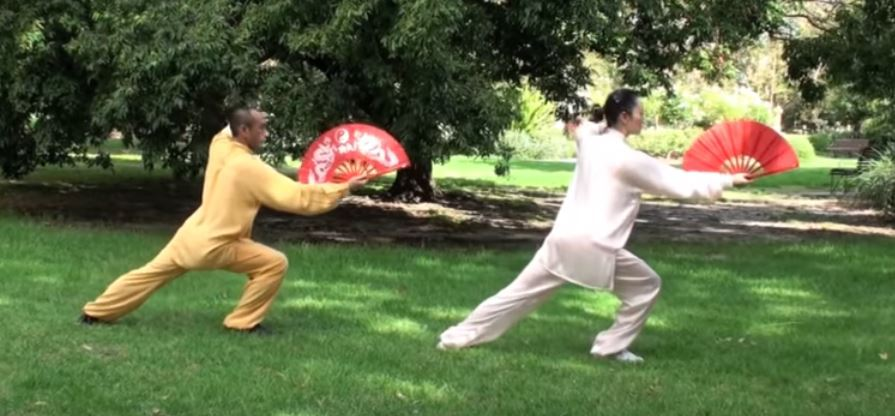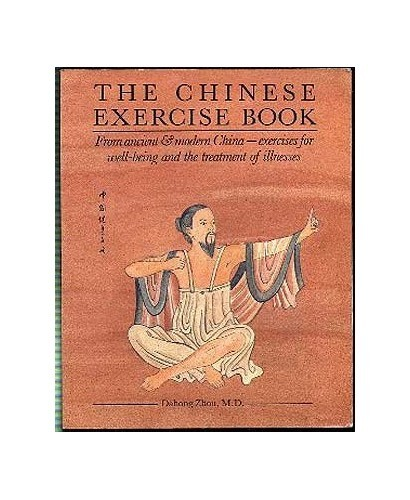Like   Tweet   Pin   +1   in/* styles */ Performance, Wellbeing, Tai Chi, Tips and Resources   ||   Issue #32 November 2020
 table div table+table+table div table{width:100%;padding:0}table div table+table+table div table img{width:96.23%;padding:0;float:none}table div table+table+table div table td{width:100%;padding:0 1.88% 18px}/* styles */Almost the end of the year and I'm sure most of us will be glad when it's over, because of the covid virus pandemic restrictions we've had to endure. Hopefully reading this November issue of Good Chi news will brighten up your day.

In this issue I share with you what I do for training before breakfast, a Tai Chi tip, an informative YouTube clip on Qigong and and another on a Tai Chi fan form. There's also a Chinese exercise book review and an ancient Lao Tzu quote.

Regards
Chris Bennett
Chris Chi

 table div table+table+table+table+table div table{width:100%;padding:0}table div table+table+table+table+table div table img{width:96.23%;padding:0;float:none}table div table+table+table+table+table div table td{width:100%;padding:0 1.88% 18px}/* styles */## Before BrekkyFollowing on from my last post in the October issue - Wake Up Call - here's a bit more about training before breakfast.

I train six days a week and always do a gentle session of either Tai Chi or Chi Kung stretches before breakfast.

Sometimes when I wake up I don't feel like training and I give myself 'a good talking to'. I always end up training and feel energised, no matter how tired I was.

Even just five to ten minutes of training is beneficial and a great way to start the day. I suggest you build up at least to a regular twenty minute session to really feel the difference.

Image courtesy of Eiliv-Sonas Aceron via Unsplash

 table div table+table+table+table+table+table+table+table div table{width:100%;padding:0}table div table+table+table+table+table+table+table+table div table img{width:96.23%;padding:0;float:none}table div table+table+table+table+table+table+table+table div table td{width:100%;padding:0 1.88% 18px}/* styles */## Tai Chi Tip

 /* styles */ Smile: Keep the corners of your mouth slightly up when practicing - helps avoid tensing your facial muscles.
 table div table+table+table+table+table+table+table+table+table+table+table div table{width:100%;padding:0}table div table+table+table+table+table+table+table+table+table+table+table div table img{width:96.23%;padding:0;float:none}table div table+table+table+table+table+table+table+table+table+table+table div table td{width:100%;padding:0 1.88% 18px}/* styles */## Understanding Qigong and QiDr. Yang Jwing-Ming gives a clear explanation on understanding Qigong and Qi in this 9.57 clip.

He says Qigong 'has been studied and practiced for over four thousand years'. The key benefits are to 'improve (your) health and cure illness'.

 table div table+table+table+table+table+table+table+table+table+table+table+table+table+table div table{width:100%;padding:0}table div table+table+table+table+table+table+table+table+table+table+table+table+table+table div table img{width:96.23%;padding:0;float:none}table div table+table+table+table+table+table+table+table+table+table+table+table+table+table div table td{width:100%;padding:0 1.88% 18px}/* styles */## Fan FormThere are many types of fan forms and here's one demonstrated by Master Liu Deming and his disciple Lele Wang.

I practice a fan form on a weekly basis and enjoy it.

It has a different flavour to the sword, sabre and spear forms I also practice.

 table div table+table+table+table+table+table+table+table+table+table+table+table+table+table+table+table+table div table{width:100%;padding:0}table div table+table+table+table+table+table+table+table+table+table+table+table+table+table+table+table+table div table img{width:96.23%;padding:0;float:none}table div table+table+table+table+table+table+table+table+table+table+table+table+table+table+table+table+table div table td{width:100%;padding:0 1.88% 18px}/* styles */## The Chinese Exercise BookThe Chinese Exercise Book by Dahong Zhou covers various gentle exercises which are ideal for beginners. The three sections in the book include traditional, fitness, and therapeutic Chinese exercises.

The illustrations and instructions are easy to follow. I've had this book in my library for several years and still refer to it when I do research on Chi Kung. The only quibble I have is that it's priced at \$54.95, which is a bit expensive if you are on a tight budget.

It's available from World of Books

 table div table+table+table+table+table+table+table+table+table+table+table+table+table+table+table+table+table+table+table+table div table{width:100%;padding:0}table div table+table+table+table+table+table+table+table+table+table+table+table+table+table+table+table+table+table+table+table div table img{width:96.23%;padding:0;float:none}table div table+table+table+table+table+table+table+table+table+table+table+table+table+table+table+table+table+table+table+table div table td{width:100%;padding:0 1.88% 18px}/* styles */table div table+table+table+table+table+table+table+table+table+table+table+table+table+table+table+table+table+table+table+table+table+table div table{width:100%;padding:0}table div table+table+table+table+table+table+table+table+table+table+table+table+table+table+table+table+table+table+table+table+table+table div table img{width:96.23%;padding:0;float:none}table div table+table+table+table+table+table+table+table+table+table+table+table+table+table+table+table+table+table+table+table+table+table div table td{width:100%;padding:0 1.88% 18px}/* styles *//* styles */
 table div table+table+table+table+table+table+table+table+table+table+table+table+table+table+table+table+table+table+table+table+table+table+table+table div table{width:100%;padding:0}table div table+table+table+table+table+table+table+table+table+table+table+table+table+table+table+table+table+table+table+table+table+table+table+table div table img{width:96.23%;padding:0;float:none}table div table+table+table+table+table+table+table+table+table+table+table+table+table+table+table+table+table+table+table+table+table+table+table+table div table td{width:100%;padding:0 1.88% 18px}/* styles *//* styles */
 table div table+table+table+table+table+table+table+table+table+table+table+table+table+table+table+table+table+table+table+table+table+table+table+table+table+table div table{width:100%;padding:0}table div table+table+table+table+table+table+table+table+table+table+table+table+table+table+table+table+table+table+table+table+table+table+table+table+table+table div table img{width:96.23%;padding:0;float:none}table div table+table+table+table+table+table+table+table+table+table+table+table+table+table+table+table+table+table+table+table+table+table+table+table+table+table div table td{width:100%;padding:0 1.88% 18px}/* styles */